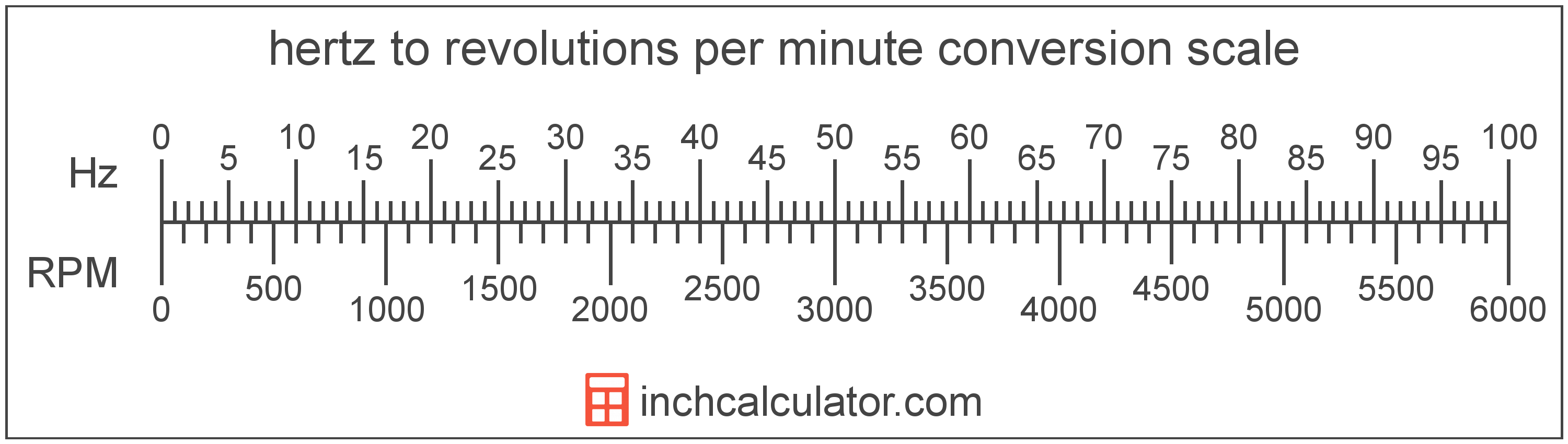Convert Revolutions Per Minute to Hertz

Enter the frequency in revolutions per minute below to get the value converted to hertz.

Results in Hertz:1 RPM = 0.016667 Hz

How to Convert Revolutions Per Minute to HertzTo convert a revolution per minute measurement to a hertz measurement, multiply the frequency by the conversion ratio. One revolution per minute is equal to 0.016667 hertz, so use this simple formula to convert:

hertz = revolutions per minute × 0.016667

The frequency in hertz is equal to the revolutions per minute multiplied by 0.016667.

For example, here's how to convert 5 revolutions per minute to hertz using the formula above.
5 RPM = (5 × 0.016667) = 0.083333 Hz

Revolutions per minute and hertz are both units used to measure frequency. Keep reading to learn more about each unit of measure.

Revolutions Per Minute

Revolutions per minute are a measure of the number of turns, or revolutions, that occur per minute.

Revolutions per minute can be abbreviated as RPM, and are also sometimes abbreviated as rev/min or r/min. For example, 1 revolution per minute can be written as 1 RPM, 1 rev/min, or 1 r/min.

Revolutions per minute can be expressed using the formula: RPM = RevolutionsTimem

Hertz

Hertz is formally defined as the frequency equalling one cycle per second.

The hertz is the SI derived unit for frequency in the metric system. Hertz can be abbreviated as Hz, for example 1 hertz can be written as 1 Hz.

Frequency in hertz can be expressed using the formula: Hz = CyclesTimes

Revolution Per Minute Measurements and Equivalent Hertz Conversions

Common revolution per minute values converted to the equivalent hertz value
Revolutions Per Minute Hertz
1 RPM 0.016667 Hz
2 RPM 0.033333 Hz
3 RPM 0.05 Hz
4 RPM 0.066667 Hz
5 RPM 0.083333 Hz
6 RPM 0.1 Hz
7 RPM 0.116667 Hz
8 RPM 0.133333 Hz
9 RPM 0.15 Hz
10 RPM 0.166667 Hz
11 RPM 0.183333 Hz
12 RPM 0.2 Hz
13 RPM 0.216667 Hz
14 RPM 0.233333 Hz
15 RPM 0.25 Hz
16 RPM 0.266667 Hz
17 RPM 0.283333 Hz
18 RPM 0.3 Hz
19 RPM 0.316667 Hz
20 RPM 0.333333 Hz
21 RPM 0.35 Hz
22 RPM 0.366667 Hz
23 RPM 0.383333 Hz
24 RPM 0.4 Hz
25 RPM 0.416667 Hz
26 RPM 0.433333 Hz
27 RPM 0.45 Hz
28 RPM 0.466667 Hz
29 RPM 0.483333 Hz
30 RPM 0.5 Hz
31 RPM 0.516667 Hz
32 RPM 0.533333 Hz
33 RPM 0.55 Hz
34 RPM 0.566667 Hz
35 RPM 0.583333 Hz
36 RPM 0.6 Hz
37 RPM 0.616667 Hz
38 RPM 0.633333 Hz
39 RPM 0.65 Hz
40 RPM 0.666667 Hz

More Revolution Per Minute Frequency Conversions

Frequency
Convert to Kilohertz
1 RPM is equal to 1.6667E-5 kilohertz
Convert to Megahertz
1 RPM is equal to 1.6667E-8 megahertz
Convert to Gigahertz
1 RPM is equal to 1.6667E-11 gigahertz
Convert to Terahertz
1 RPM is equal to 1.6667E-14 terahertz
Angular Frequency
Convert to Cycles Per Second
1 RPM is equal to 0.016667 cycles per second
Convert to Radians Per Second
1 RPM is equal to 0.10472 radians per second
Convert to Degrees Per Second
1 RPM is equal to 6 degrees per second